# High School Math : How to find the volume of a cone

## Example Questions

2 Next →

### Example Question #11 : Solid Geometry

What is the volume of a cone with base radius 4, and height 6?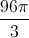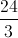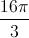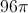Explanation:

The volume of a cone is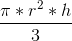, whereis the height of the cone andis the base radius.

The volume of this cone is thus: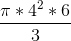=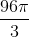2 Next →